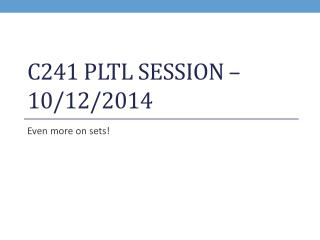DownloadDownload PresentationC241 PLTL Session – 10/12/2014

# C241 PLTL Session – 10/12/2014

Télécharger la présentation## C241 PLTL Session – 10/12/2014

- - - - - - - - - - - - - - - - - - - - - - - - - - - E N D - - - - - - - - - - - - - - - - - - - - - - - - - - -
##### Presentation Transcript

1. C241 PLTL Session – 10/12/2014 Even more on sets!

2. Warm-Up Exercise • Grab a worksheet • Begin completing the worksheet in pairs or small groups

3. Problem 1 How many elements are in a set within a set?

4. Problem 2 What is the set difference between (3, 4, 6, 2, 1) and ((), (3, 4, 6, 2, 1), 3, 2, 1)?

5. Problem 3 Visit the SETL website, http://setl.org/setl-server.html, and answer the following questions: (Give both the answer and the SETL code that generated you this answer.) Note that sets are denoted by braces {}, the intersection operator for sets is denoted by *, and the union operator for sets is denoted + in SETL. Using parentheses, (), to denote a set is a more conventional notation than using braces, {}. A = (11, 4, 13, 4, 25, 6, (), (1, 2)), B = (1, 4, 25, 6, (), 17, 8, 9), and C = (4, 7, 8, 9, 10, (11, 12, 13), 12) (i) What is the set difference between A and C? (ii) What is the set difference between C and D = ((11, 12, 13))?

6. Problem 4 from last week’s PowerPoint Visit the SETL website, http://setl.org/setl-server.html, and answer the following questions: (Give both the answer and the SETL code that generated you this answer.) Note that sets are denoted by braces {}, the intersection operator for sets is denoted by *, and the union operator for sets is denoted + in SETL. Using parentheses, (), to denote a set is a more conventional notation than using braces, {}. A = (11, 4, 13, 4, 25, 6, (), (1, 2)), B = (1, 4, 25, 6, (), 17, 8, 9), and C = (4, 7, 8, 9, 10, (11, 12, 13), 12) (i) What is the set difference between A and B? (ii) What is the set difference between C and D = (11, 12, 13)?